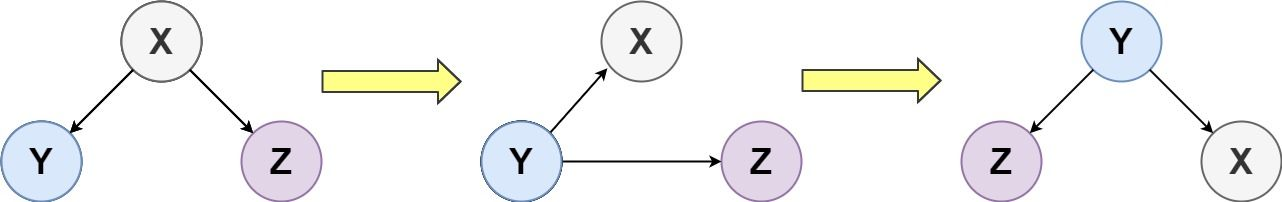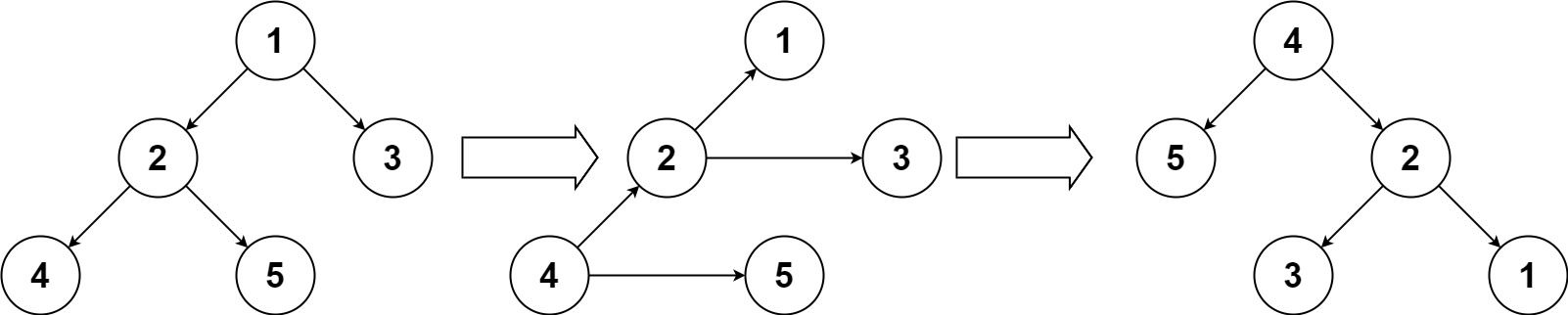# Question

Formatted question description: https://leetcode.ca/all/156.html

Given the root of a binary tree, turn the tree upside down and return the new root.

You can turn a binary tree upside down with the following steps:

1. The original left child becomes the new root.
2. The original root becomes the new right child.
3. The original right child becomes the new left child.The mentioned steps are done level by level. It is guaranteed that every right node has a sibling (a left node with the same parent) and has no children.

Example 1:Input: root = [1,2,3,4,5]
Output: [4,5,2,null,null,3,1]


Example 2:

Input: root = []
Output: []


Example 3:

Input: root = 
Output: 


Constraints:

• The number of nodes in the tree will be in the range [0, 10].
• 1 <= Node.val <= 10
• Every right node in the tree has a sibling (a left node that shares the same parent).
• Every right node in the tree has no children.

# Algorithm

To flip a binary tree, we start with a root node and aim to turn its left child node into a root node, and its right child node into a left child node, while the original root node becomes a right child node. We can perform this operation recursively as follows:

• First, we check if the root node exists and has a left child node. If not, we return directly without any operation.

• Next, we call the flip function recursively on the left child node until we reach the leftmost child node.

• Once we reach the leftmost child node, we start flipping the nodes, and then return to the previous left child node and continue flipping until the complete tree is flipped.

During the flipping process, we swap the left and right child nodes of each node starting from the leftmost child node up to the root node. We can represent the left child node as node.left and the right child node as node.right. So, the flipping operation can be written as:

node.left, node.right = node.right, node.left


By applying this operation recursively, we can flip the entire binary tree.

# Code

• 
public class Binary_Tree_Upside_Down {
/**
* Definition for a binary tree node.
* public class TreeNode {
*     int val;
*     TreeNode left;
*     TreeNode right;
*     TreeNode(int x) { val = x; }
* }
*/
public class Solution {
public TreeNode upsideDownBinaryTree(TreeNode root) {

if (root == null) {
return root;
}

return dfs(root, null);
}

private TreeNode dfs(TreeNode root, TreeNode parent) {

if (root == null) {
return root;
}

TreeNode newRoot = dfs(parent.left, root);
newRoot.left = parent == null ? null : parent.right;
newRoot.right = parent;

return newRoot;
}
}

public class Solution_iteration {
public TreeNode upsideDownBinaryTree(TreeNode root) {

if (root == null) {
return root;
}

TreeNode current = root;
TreeNode prev = null;
TreeNode next = null;
TreeNode tmp = null;

while (current != null) {
next = current.left;
current.left = tmp;
tmp = current.right;
current.right = prev;
prev = current;
current = next;
}

return prev;
}
}
}

############

/**
* Definition for a binary tree node.
* public class TreeNode {
*     int val;
*     TreeNode left;
*     TreeNode right;
*     TreeNode() {}
*     TreeNode(int val) { this.val = val; }
*     TreeNode(int val, TreeNode left, TreeNode right) {
*         this.val = val;
*         this.left = left;
*         this.right = right;
*     }
* }
*/
class Solution {
public TreeNode upsideDownBinaryTree(TreeNode root) {
if (root == null || root.left == null) {
return root;
}
TreeNode newRoot = upsideDownBinaryTree(root.left);
root.left.right = root;
root.left.left = root.right;
root.left = null;
root.right = null;
return newRoot;
}
}

• // OJ: https://leetcode.com/problems/binary-tree-upside-down/
// Time: O(N)
// Space: O(1)
class Solution {
public:
TreeNode* upsideDownBinaryTree(TreeNode* root) {
if (!root || !root->left) return root;
TreeNode *left = root->left, *right = root->right, *newRoot = NULL;
while (left) {
TreeNode *nextLeft = left->left, *nextRight = left->right;
if (!newRoot) root->left = root->right = NULL;
newRoot = left;
newRoot->left = right;
newRoot->right = root;
root = left;
left = nextLeft;
right = nextRight;
}
return newRoot;
}
};

• # Definition for a binary tree node.
# class TreeNode:
#     def __init__(self, val=0, left=None, right=None):
#         self.val = val
#         self.left = left
#         self.right = right
class Solution:
def upsideDownBinaryTree(self, root: Optional[TreeNode]) -> Optional[TreeNode]:
if root is None or root.left is None:
return root
new_root = self.upsideDownBinaryTree(root.left)
root.left.right = root
root.left.left = root.right
root.left = None
root.right = None
return new_root

############

# Definition for a binary tree node.
# class TreeNode(object):
#     def __init__(self, x):
#         self.val = x
#         self.left = None
#         self.right = None

class Solution(object):
def upsideDownBinaryTree(self, root):
"""
:type root: TreeNode
:rtype: TreeNode
"""
if not root:
return

left = self.upsideDownBinaryTree(root.left)
right = self.upsideDownBinaryTree(root.right)
if root.left:
root.left.right = root
root.left.left = root.right
root.left = root.right = None
return left if left else root


• /**
* Definition for a binary tree node.
* type TreeNode struct {
*     Val int
*     Left *TreeNode
*     Right *TreeNode
* }
*/
func upsideDownBinaryTree(root *TreeNode) *TreeNode {
if root == nil || root.Left == nil {
return root
}
newRoot := upsideDownBinaryTree(root.Left)
root.Left.Right = root
root.Left.Left = root.Right
root.Left = nil
root.Right = nil
return newRoot
}